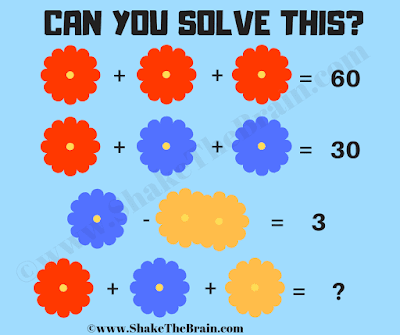This is a very interesting flower math picture puzzle. In this math picture puzzle, you are shown some mathematical algebraic equations. Variables in these fun math equations are represented by flowers. Your challenge is to find the value of each given flower and then solve the last equation to find the value of the missing number.Can you solve this math picture puzzle?
Answer of this "Flowers Math Picture Puzzle", can be viewed by clicking on the answer button.

Unknown said...

26